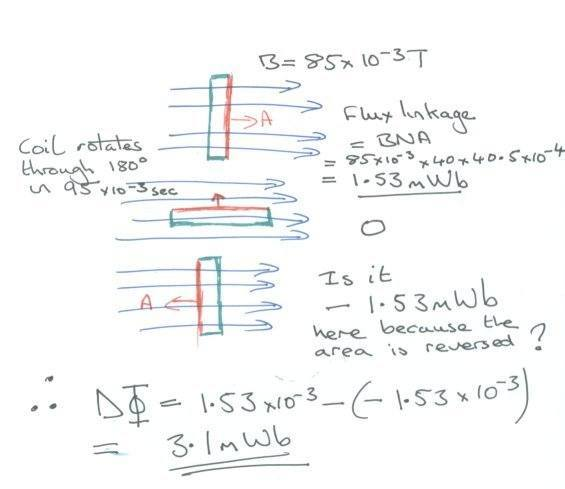# Magnetic flux linkage and area as a vector

## Homework Statement

A circular coil of diameter 24 mm has 40 turns. The coil is placed in a uniform magnetic field of flux density 85 mT with its plane perpendicular to the field lines.

a) i Calculate the area of the coil

ii The flux linkage through the coil.

b) The coil was reversed in a time of 95 ms.

Calculate

i the change of flux linkage through the coil.

ii the magnitude of the induced emf

## Homework Equations

EMF = rate of change of flux linkage

## The Attempt at a Solution

Part a I am ok with ai) area = 4.5x10^-4 and flux linkage is BNA = 1.53 mWb
In part b I struggled to see why the answer was 3.06 mWb and not a change of 1.53 to zero as it rotated.
my logic being that it changes from 1.53 to zero as it rotated for the first 90 degrees then back to 1.53.

I remember reading area is a vector so if this is true does it mean the flux linkage is changing from 1.53 mWb to -1.53 mWb, This would give me the correct answer in the back of the text book.

Is there anyway of seeing this without knowing that area is a vector as this is not in the text book as a principle taught on my course.

Thanks you for any help
Jimmy